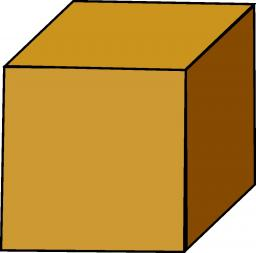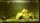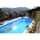# Hectoliter into cube

Can 1hl of water fit into a half-meter cube?

Result

V=

#### Solution:We would be pleased if you find an error in the word problem, spelling mistakes, or inaccuracies and send it to us. Thank you!Tips to related online calculators
Do you know the volume and unit volume, and want to convert volume units?

## Next similar math problems:

• Swimming poolThe swimming pool has the shape of a block with dimensions of 70dm, 25m, 200cm. How many hl of water can fit into the pool?
• Water tank300hl of water was filled into the tank 12 m long and 6 m wide. How high does it reach?
• Water reservoirThe water tank has a cuboid with edges a= 1 m, b=2 m , c = 1 m. Calculate how many centimeters of water level falls, if we fill fifteen 12 liters cans.
• Cylindrical tank9.6 hl of water is poured into a cylindrical tank with a bottom diameter of 1.2 m. What height in centimeters does the water reach?
• Cube and waterHow many liters of water can fit into a cube with an edge length of 0.11 m?
• WaterInto a full cylindrical tank high 3 with base radius 2.4 m we insert cuboid with dimensions 1.7 m, 1.7 m, 0.8 m. How many liters of water will come out?
• HectolitersHow many hectoliters of water fits into cuboid tank with dimensions of a = 3.5 m b = 2.5 m c = 1.4 m?
• AquariumHow many liters of water can fit in an aquarium measuring 30, 15 and 20 cm?
• RainfallThe annual average rainfall in India was in Cherrapunji in the year 1981 26 461 mm. How many hectoliters of water fell on 1 m2? Would fit this amount of water into a cube of three meters?
• AquariumAquarium is cube with edge 45 cm. How much water can fit in there?
• Two cuboidsFind the volume of cuboidal box whose one edge is: a) 1.4m and b) 2.1dm
• TankThe tank bottom has dimensions of 1.5 m and 3 2/6 m. The tank is 459.1 hl of water. How high is the water surface?
• AquariumCan 30 liters of water fit in a cuboid aquarium with dimensions a = 3dm b = 6dm c = 5dm?
• Water poolWhat water level is in the pool shaped cuboid with bottom dimensions of 25 m and 10 meters, when is 3750hl water in the pool.
• Cubes into cuboidHow many 12 centimeter cubes fit into the block (cuboid) with 6dm, 8.4dm, and 4.8?
• Three-quarters of its volumeThe pool has a block shape with a length of 8m, a width of 5.3m and a depth of 1.5m. How many hl of water is in it if it is filled to three-quarters of its volume?
• Cylinder containerThe cylindrical container with a diameter of 1.8 m contains 2,000 liters of water. How high does the water reach?